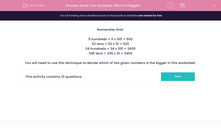# Know Your Numbers: Which is Bigger?

In this worksheet, students decide which of two numbers given in different formats is bigger.This content is premium and exclusive to EdPlace subscribers.Key stage:  KS 2

Curriculum topic:   Maths and Numerical Reasoning

Curriculum subtopic:   Place Value

Difficulty level:#### Worksheet Overview

Remember that:

5 hundreds = 5 x 100 = 500

52 tens = 52 x 10 = 520

34 hundreds = 34 x 100 = 3400

345 tens = 345 x 10 = 3450

You will need to use this technique to decide which of two given numbers is the bigger in this worksheet.

### What is EdPlace?

We're your National Curriculum aligned online education content provider helping each child succeed in English, maths and science from year 1 to GCSE. With an EdPlace account you’ll be able to track and measure progress, helping each child achieve their best. We build confidence and attainment by personalising each child’s learning at a level that suits them.

Get started